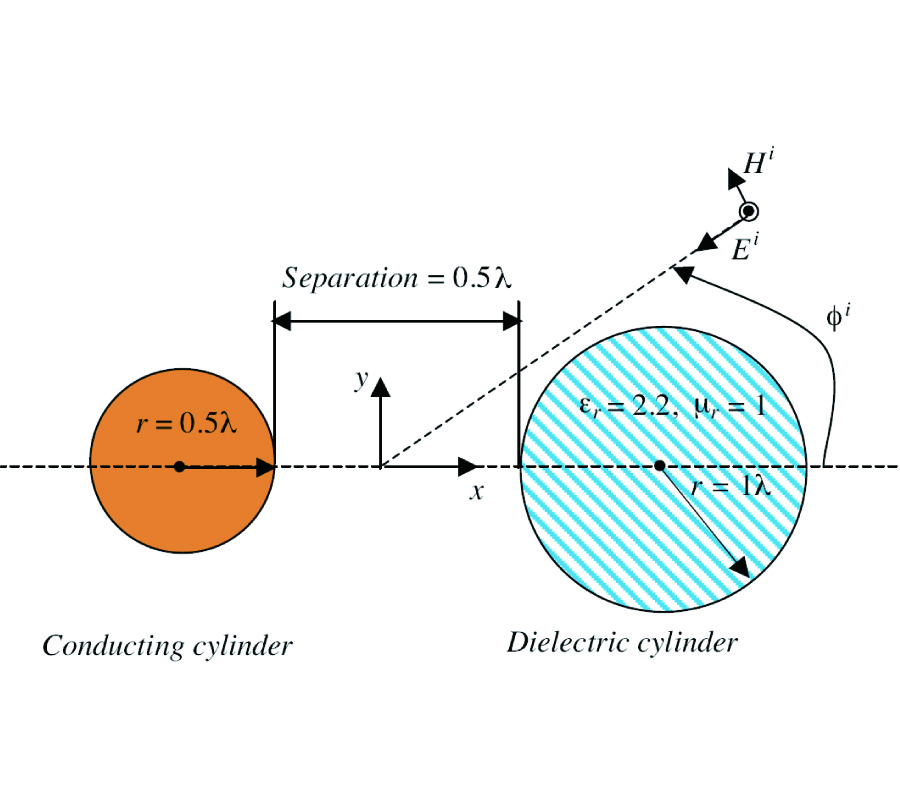Vol. 57
Latest Volume
All Volumes
All Journals
2005-11-01
PIER
Vol. 57, 237-252, 2006
A Parallelized 3D Floating Random-Walk Algorithm for the Solution of the Nonlinear Poisson-Boltzmann Equation
Kausik Chatterjee and Jonathan Poggie
This paper presents a new three-dimensional floating random-walk (FRW) algorithm for the solution of the Nonlinear Poisson-Boltzmann (NPB) equation. The FRW method has not been previously used in the numerical solution of the NPB equation (and other nonlinear equations) because of the non-availability of analytical expressions for volumetric Green's functions. In the past, numerical studies using the FRW method have examined only the linearized Poisson-Boltzmann equation, producing solutions that are only accurate for small values of the potential. No such linearization is required for this algorithm. An approximate expression for a volumetric Green's functions has been calculated with the help of a novel use of perturbation theory, and the resultant integral form has been incorporated within the FRW framework. The algorithm requires no discretization of either the volume or the surface of the problem domains, and hence the memory requirements are expected to be lower than approaches based on spatial discretization, such as finite-difference methods. Another advantage of this algorithm is that each random walk is independent, so that the computational procedure is inherently parallelizable and an almost linear increase in computational speed is expected with increase in the number of processors. We have recently published the preliminary results for benchmark problems in one and two dimensions. In this work, we present our results for benchmark problems in three dimensions and demonstrate excellent agreement between the FRW- and finite-difference based algorithms. We also present the results of parallelization of the newly developed FRW algorithm. The solution of the NPB equation has applications in diverse branches of science and engineering including (but not limited to) the modeling of plasma discharges, semiconductor device modeling and the modeling of biomolecular structures and dynamics.2005-10-07
PIER
Vol. 57, 253-264, 2006
Full-Wave Modeling of Stripline Structures in Multilayer Dielectrics
Konstantinos Nikellis , Nikolaos Uzunoglu , Yorgos Koutsoyannopoulos and Sotiris Bantas
A novel computational method based on full-wave analysis of stripline planar structures with vertical interconnects in multilayer dielectric media is presented. The method is based on the electric-field integral-equation solved with the Method of Moments (MoM). The special characteristics of stripline structures facilitate the extensive use of semi-analytical techniques to analyze the multilayer structures, limiting significantly the use of purely numerical techniques. The accuracy of the proposed modeling method is examined thoroughly with extensive numerical tests and the results are compared with results generated by commercial simulators for simple stripline structures.2005-09-27
PIER
Vol. 57, 311-333, 2006
Applying Divergence-Free Condition in Solving the Volume Integral Equation
Maokun Li and Weng Cho Chew
Applying divergence-free condition to volume integral equation solver will be discussed. Three schemes are available: basis reduction scheme, minimal complete volume loop basis set and expanded volume loop basis set. All of them will generate smaller matrix equations than the SWG basis. The first two schemes generate poorly-conditioned matrices that are hard to solve by iterative solvers. The expanded loop basis set is easier to solve iteratively in spite of the existence of a null space in the matrix. Moreover, the construction of the expanded loop basis set is much easier than the other two schemes.2005-09-27
PIER
Vol. 57, 285-310, 2006
Mixture Effective Permittivity Simulations Using Imlmqrf Method on Preconditioned EFIE
Hao-Gang Wang and Chi Chan
Effective permittivity of mixtures of lossy dielectric are important quantities to be studied in microwave remote sensing of soil moisture, sea ice, dry and wet snow, in geophysical probing of properties of porous rocks and in composite materials. In this paper, these quantities are studied with large-scale numerical solutions of Maxwell equations in the electroquasistatic limit using fast electromagnetic algorithms. The preconditioned EFIE method instead of scalar potential scattering method for the simulation of the relative permittivity of the mixture with conducting particles in quasi-static environment is introduced. Furthermore, Algorithm IMLMQRF, which is kernel independent for quasi-static problems with a complexity of O(N log N), is implemented to accelerate the matrix-vector multiply when CG iteration is applying on this preconditioned EFIE system. Subsequently, numerical examples, viz., the permittivity extractions from two lattice structures and one random distribution structure with the unknowns from about 1,000 to 50,000 are efficiently simulated by our method in this paper. The numerical results demonstrate the efficiency of this hybrid method.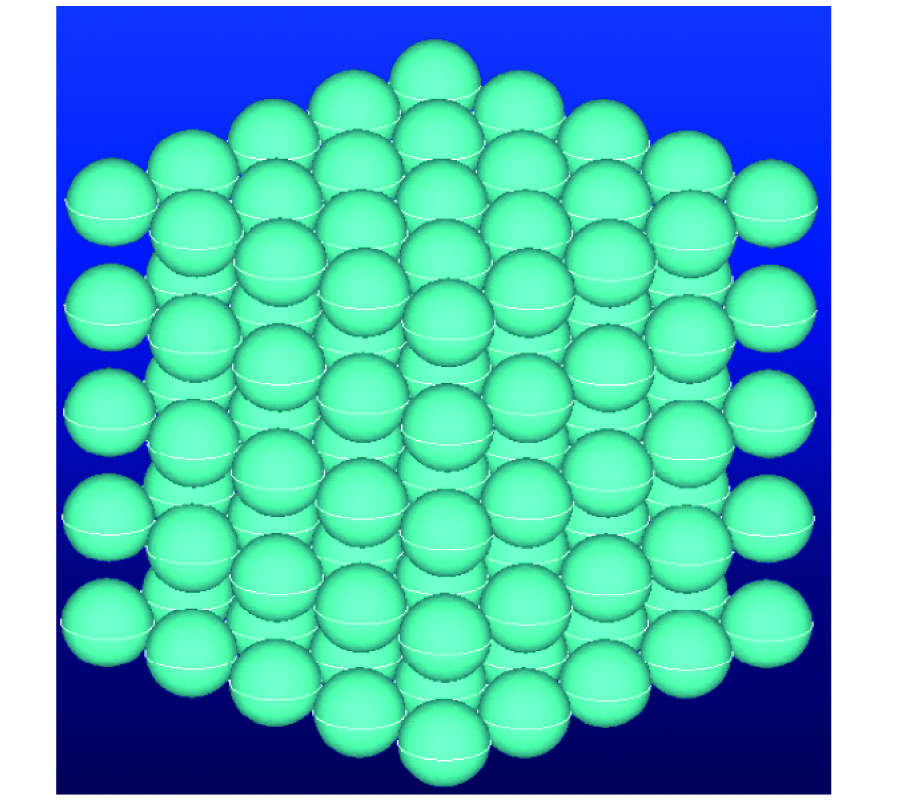2005-09-27
PIER
Vol. 57, 277-284, 2006
Analysis of Human Semen Using Microwaves
Anil Lonappan , Gopinathan Nair Bindu , Vinu Thomas and K. Mathew
Microwave engineering now a days plays a vital tool in diagnostic and therapeutic medicine. A quality evaluation of human semen at microwave frequencies using the measurements made at different intervals of time by cavity perturbation technique in the S-band of microwave spectrum is presented in this paper. Semen samples were also examined in the microscopic as well as macroscopic level in clinical laboratory. It is observed that conductivity of semen depends upon the motility of sperm and it increases as time elapses, which finds applications in forensic medicine.2005-09-27
PIER
Vol. 57, 265-276, 2006
RCS Computation of Airplane Using Parabolic Equation
The accurate analysis of scattering from ob jects with dimensions large compared to the wavelength using rigorous methods (finite element, FDTD, method of moments) with a personal computer is almost impractical. In asymptotic methods, physical optics (PO), geometrical theory of diffraction (GTD), the accurate modeling of the ob ject's boundary is too cumbersome. The parabolic equation method gives accurate results in calculation of scattering from ob jects with dimensions ranging from one to tens of wavelengths. Solving parabolic equation with the marching method needs limited computer storage even for scattering calculations of large targets. In this paper, first the calculation procedure of radar cross section using parabolic equation in three dimension is studied and the necessary equations are derived. In order to show the validity of the parabolic equation, the RCS of a conducting sphere is calculated and the results are compared with analytic results. The airplane RCS has been computed by using a staircase model in the parabolic equation and the results are compared with physical optics results.2005-09-27
PIER
Vol. 57, 209-236, 2006
Spherical Waves in Conical TEM Cells
David Pouhe
The propagation of the TEM and higher order Modes in GTEM cells is theoretically treated in this work in spherical coordinates. The derived wave equation for the principal mode is solved analytically in two different ways. In which case, two general closed-solutions are derived. In addition to this a simple approximation for the special case of the symmetric cells is presented. For higher order waves, E- and H-modes are determined by solving the Helmholz equations for phasor. By imposing the boundary conditions on fields, the determination of local higher-order modes is, for symmetric cells, reduced to the solution of a simple transcendental equation Lmνn (cos θ) = 0. For asymmetric cells, the matching-points method is applied. In the longitudinal direction, the propagation of the fields is examined by means of cylindrical functions which are combined with the spherical one. Furthermore, since the GTEM cell is a conical-horn resonator, the resonance behavior of the cell is investigated. The main advantages of the method amongst others are its simplicity and high degree of accuracy. Its appeal consist of precise description of the cell's geometry compared with the other methods.2005-09-27
PIER
Vol. 57, 197-208, 2006
A Modified Three-Antenna Gain Measurement Method to Simplify Uncertainty Estimation
Krishnasamy Selvan
A modified three-antenna gain measurement method is proposed to significantly simplify, and thereby to reduce the costs of, uncertainty estimation. Unlike the three-antenna method, the new method employs a standard antenna whose gains and uncertainty limits have been established through absolute calibration. The measurements are carried out as in the three-antenna method, with the reference antenna also analysed as presumed unknown. An overall uncertainty figure for the measurement is obtained only from the resulting data in conjunction with the uncertainty on the reference antenna gain values. The proposal is verified by using a rigorous experimental study on the gains of three pyramidal horns in the frequency range 5.8 GHz-8.2 GHz. First, the gains and error limits of the antennas are estimated by using the three-antenna method. One of the antennas is then used as reference antenna and the modified method employed on the measured data to determine the gains of a test antenna. It emerges that the new method substantially simplifies the secondary calibration and gain measurement of antennas.
2005-09-27
PIER
Vol. 57, 179-195, 2006
Effect of Round Corners on Bowtie Antennas
Shi-Wei Qu and Cheng-Li Ruan
In this paper, three types of novel bowtie antennas with round corners are presented and studied carefully, including quadrate, rounded-edge and triangular shapes, which have better return loss, flatter input impedance, more stable radiation patterns and smaller area at the same time. The effect of round corners is attached importance to due to their novelty. The conclusion is drawn that adding the round corners at the sharp vertexes of radiation surfaces can have positive effects on performances of not only bowtie antennas but also the others.2005-09-27
PIER
Vol. 57, 165-177, 2006
Electromagnetic Scattering by Parallel Metamaterial Cylinders
Alireza Shooshtari and Abdel Sebak
Electromagnetic Scattering problem by multiple metamaterial cylinders are studied. A formulation for coating conducting cylinders by metamaterial is presented. The formulation is based on the expansion of electromagnetic fields by Bessel functions in local cylindrical coordinates and then transformation from local coordinates to others using addition theorem of Hankel function. Near fields and scattering cross sections for metamaterial and dielectric cylinders are studied and compared to each other. We have shown that an array of metamaterial cylinders increases forward scattering cross section which may use to increase the directivity of antennas.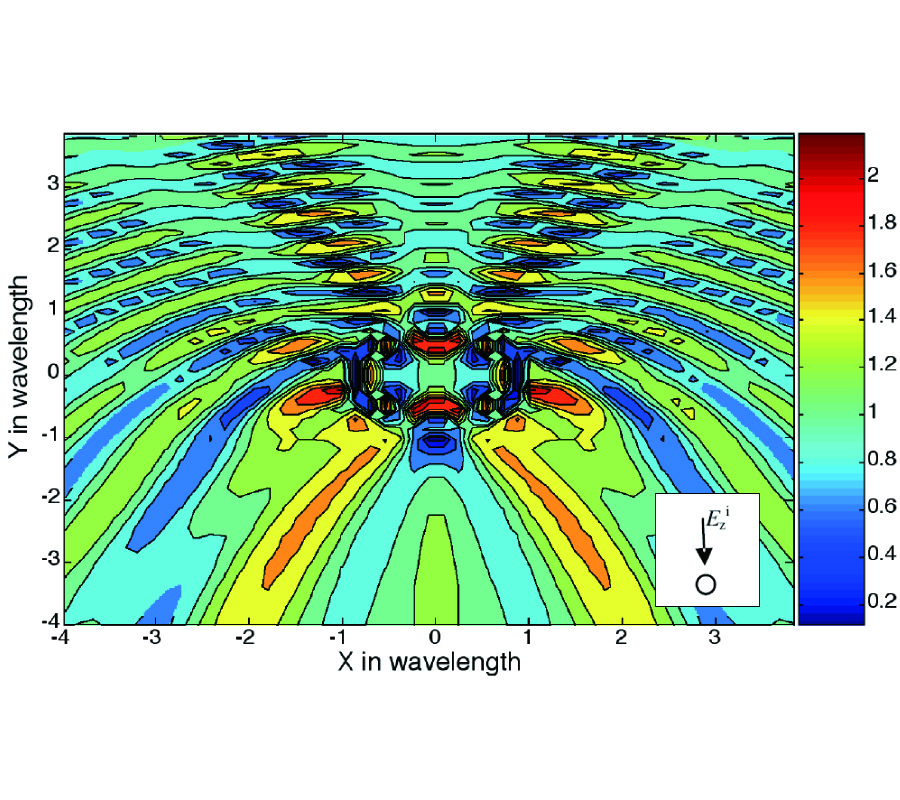2005-09-27
PIER
Vol. 57, 151-163, 2006
Scattering Computation from the Target with Lossy Background
The accurate analysis of scattering from ob jects with dimensions large compared to the wavelength using rigorous methods (finite element, FDTD, method of moments) with a personal computer is almost impractical. In asymptotic methods, physical optics (PO), geometrical theory of diffraction (GTD), the accurate modeling of the object's boundary is too cumbersome. The parabolic equation method gives accurate results in calculation of scattering from objects with dimensions ranging from one to tens of wavelengths. Solving parabolic equation with the marching method needs limited computer storage even for scattering calculations of large targets. In this paper, first the calculation procedure of radar cross section using parabolic equation is studied and the necessary equations are derived. The parabolic equation and the model of reflecting facet is utilized for calculation of the scattered fields in the forward and backward directions. In order to model the lossy background, the impedance boundary condition is utilized in lower boundary. Finally the scattered fields and RCS of a ship and a tank are calculated as two examples of targets with lossy background.2005-09-27
PIER
Vol. 57, 131-150, 2006
Radiation from Dielectric Coated Elliptic Conducting Cylinder by Assigned Electric Current Distribution
Giovanni Angiulli , Giandomenico Amendola and Giuseppe Di Massa
In this paper the analysis of radiation from known current distributions placed on a dielectric coated elliptic conducting cylinder has been effected. The analysis is carried out considering the expansion of the field as a series of Mathieu functions. The presence of the current is taken into account in the boundary conditions at the air-dielectric interface. The behavior of the radiated field as a function of the minor over ma jor axis ratio of the elliptic conducting cylinder has been investigated and discussed.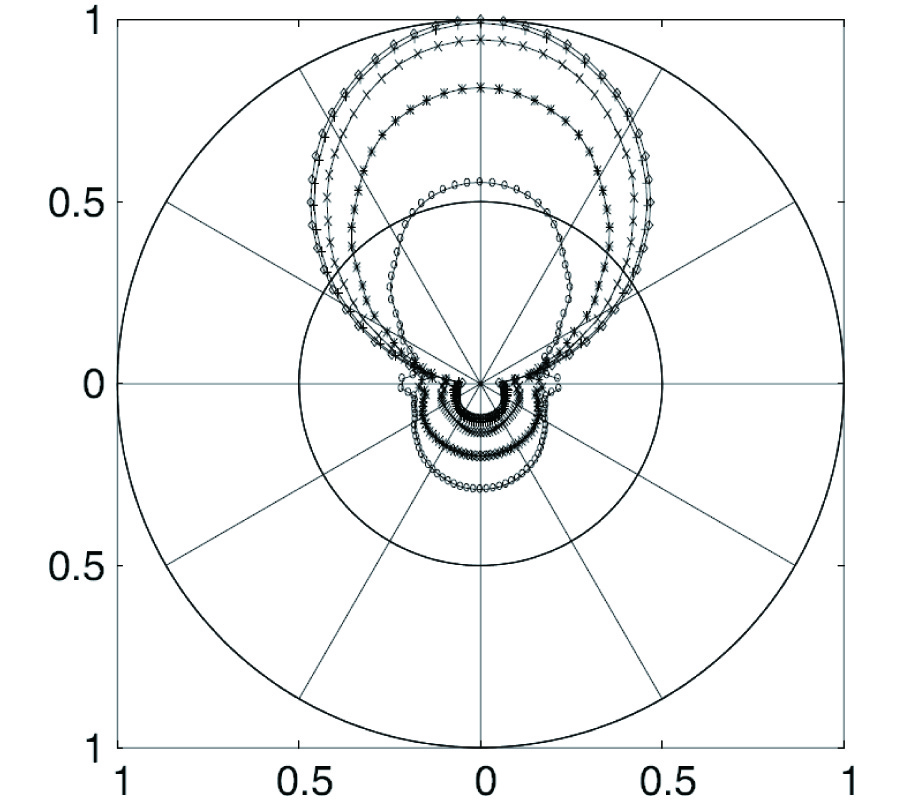2005-09-27
PIER
Vol. 57, 101-129, 2006
The Asymptotic Solution of an Integral Equation for Magnetic Current in a Problem of Waveguides Coupling through Narrow Slots
Mikhail Nesterenko and V. Katrich
Based on the asymptotic method of averaging, an approximate analytical solution of the integral equation concerning a magnetic current in slot-hole coupling apertures of electrodynamic volumes, which differ profitably from the known ones in literature, has been obtained. The formulas for the currents and characteristics scattering of transverse and longitudinal slots in common broad and narrow walls of rectangular waveguides are given. The comparison to results obtained by other methods and experimental data has been done.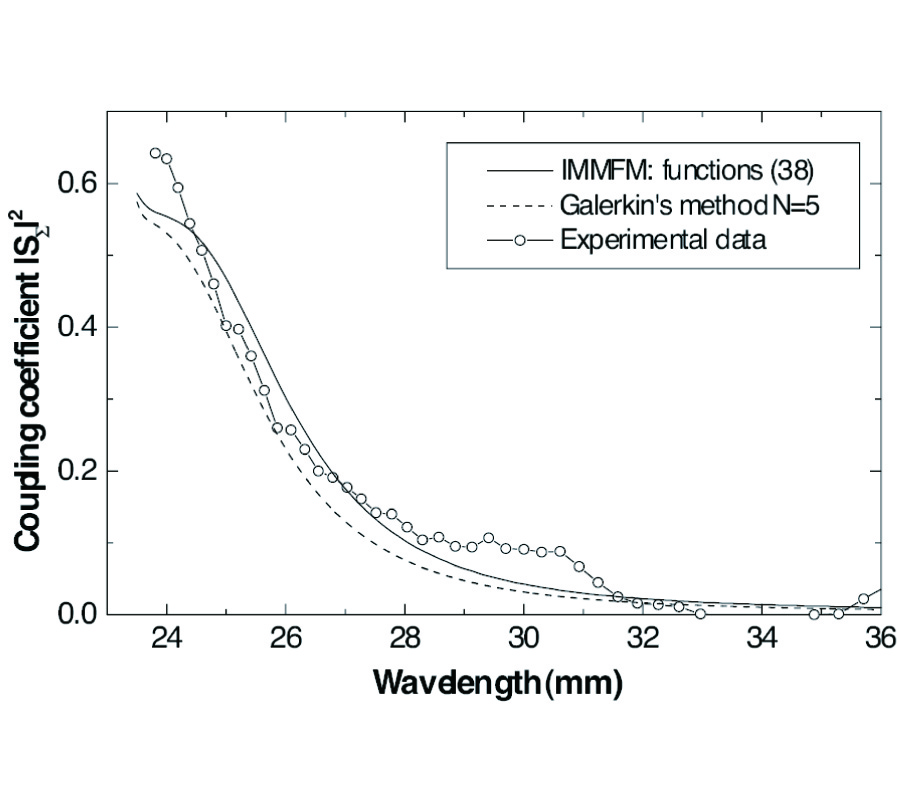2005-09-27
PIER
Vol. 57, 85-100, 2006
A Novel Marching Algorithm for Radio Wave Propagation Modeling Over Rough Surfaces
In this paper, the parabolic approximation of wave equation will be solved by the method of least squares. At first, the radio wave propagation in homogeneous media will be considered. The electromagnetic field will be expanded by proper expansion functions, which satisfy the parabolic equation in homogeneous media. The expansion coefficients will be derived by the least square method through enforcing initial and boundary conditions. The least square functionals satisfy the initial and boundary conditions. Similar to the split step method, the field in the inhomogeneous media with known profile of refractive index can be obtained by proper phase shifting of the field in homogeneous media. The proposed method is more reliable than the split step method and can be applied over rough boundary without any excess computations. In comparison with the finite difference method, the proposed method is very fast.2005-09-27
PIER
Vol. 57, 55-84, 2006
Electromagnetic Scattering by a Set of Objects: an Integral Method Based on Scattering Operator
Daniel Maystre
The paper presents the Scattering Operator Method, which is devoted to the problem of scattering from a set of N cylindrical ob jects. By contrast with the Scattering Matrix Method, which has been used by many groups in the last twenty years, it applies to any kind of cylinder shape, regardless of the relative location of the cylinders. The theory is based on a mathematical result: it is possible to define in the vicinity of the surface of each cylinder two complementary parts of the field: the total incident field and the field scattered by this cylinder. These two parts are the Calderon projectors of the values of the total fields on the surface of the cylinder. The validity of the method is checked on two examples. It is shown that the theory avoids some problems encountered in integral method like evaluations of singular or hypersingular integrals, or instabilities due to internal resonance of ob jects.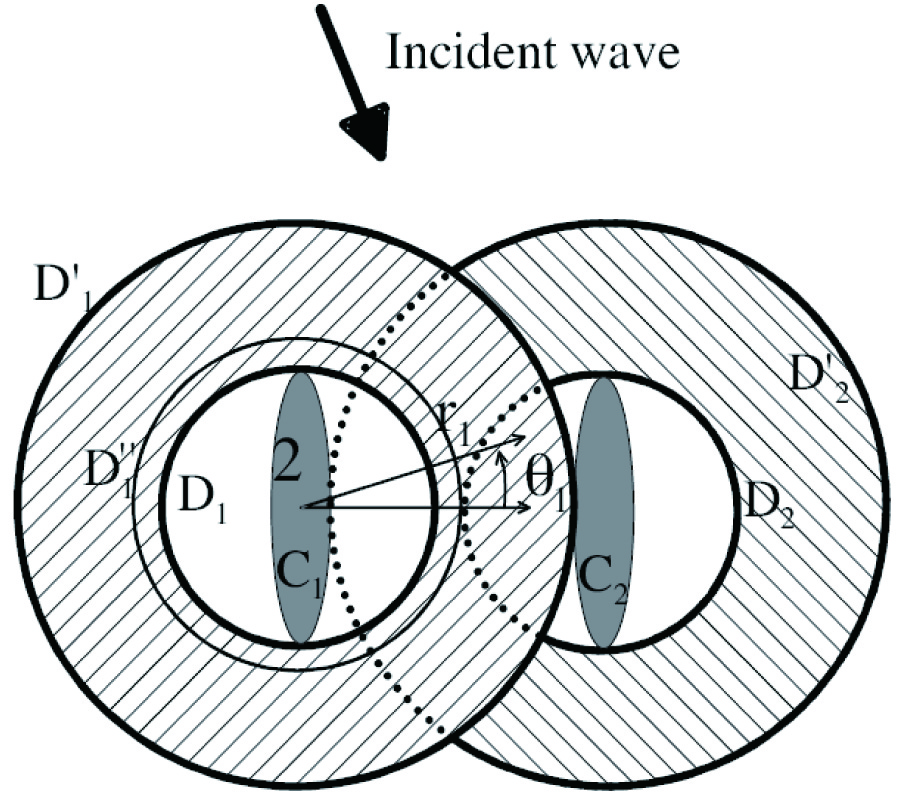2005-09-27
PIER
Vol. 57, 33-54, 2006
Field Structures of Waveguide Junction Circulators with Irregular Shaped Ferrite Simulated Based on Exact Treatment
H. Wu and Wen-Bin Dou
Field structures of waveguide Y-junction circulators containing irregular shaped ferrite material, such as Y-junction with partial-height cylinder/triangle ferrite posts and metal step impedance transformer, Y-junction with a ferrite sphere, and dual higher order modes circulator, which are classified as different junction resonance modes, are depicted to illustrate the circulating process. The analysis method used is a combination of the mode expansion method and finite element method, which is based on the weak form of Helmholtz equation. Calculated results (return loss, insertion loss and isolation) of these structures are shown and compared respectively to those in literatures. Consistencies have been observed.2005-09-27
PIER
Vol. 57, 19-32, 2006